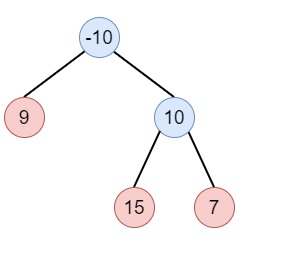# Binary Tree Maximum Path Sum in Python

Suppose we have one non-empty binary tree. We have to find the path sum. So here, a path is any sequence of nodes from some starting node to any node in the where the parent-child connections are present. The path must contain at least one node and does not need to go through the root node. So if the input tree is −Here the output will be 32.

To solve this, we will follow these steps −

• Define one method called solve(), this will take node

• if node is null or the value of node is 0, then return 0

• left := max of 0 and solve(left of node)

• right := max of 0 and solve(right of node)

• ans := max of ans and left + right + data of the node

• return node data + max of left and right

• From the main method, set ans := -inf, then call solve(root) and return ans

## Example

Let us see the following implementation to get better understanding −

Live Demo

class TreeNode:
def __init__(self, data, left = None, right = None):
self.data = data
self.left = left
self.right = right
def insert(temp,data):
que = []
que.append(temp)
while (len(que)):
temp = que
que.pop(0)
if (not temp.left):
if data is not None:
temp.left = TreeNode(data)
else:
temp.left = TreeNode(0)
break
else:
que.append(temp.left)
if (not temp.right):
if data is not None:
temp.right = TreeNode(data)
else:
temp.right = TreeNode(0)
break
else:
que.append(temp.right)
def make_tree(elements):
Tree = TreeNode(elements)
for element in elements[1:]:
insert(Tree, element)
return Tree
class Solution(object):
def maxPathSum(self, root):
self.ans = -float('inf')
self.solve(root)
return self.ans
def solve(self,node):
if not node or node.data == 0:
return 0
left = max(0,self.solve(node.left))
right = max(0,self.solve(node.right))
self.ans = max(self.ans,left+right+node.data)
return node.data + max(left,right)
ob = Solution()
root = make_tree([-10,9,10,None,None,15,7])
print(ob.maxPathSum(root))

## Input

[-10,9,10,None,None,15,7]

## Output

32